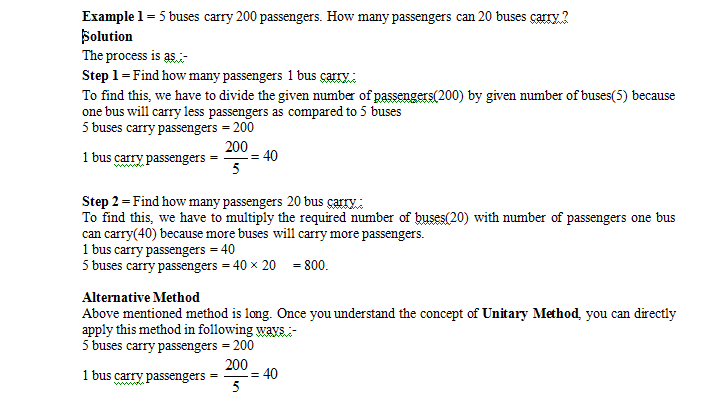# Proportion

## Comparing Quantities of Class 7

In the simple word we can Proportion as the equality of ratios is called “proportion”.

If a : b :: c : d then a, b, c and d are said to be in proportion. Then a, b, c and d are called terms.

The first and fourth i.e. a and d are called extremes and second and third term i.e. a and d are called mean terms and d is called the fourth proportional.

Product of Means = Product of Extremes

If a:b = c:d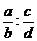Then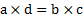If four quantities be in proportion, the product of the extremes is equal to the product of the means.

In Proportion we have to know different types of proportion a

DIRECT PROPORTION (Direct Variation) –

If two quantities are linked in such a way that an increase in one quantity lead to a corresponding increase in the other and vice-versa, then such a variation is called direct variation.

Ex.If 10 calculators cost Rs120, how much will 8 calculators cost?

To solve this, we need to change "10 chocolate" into "8 chocolate".

First we start by finding out what 1 chocolate costs, by dividing both parts of our fact by 10:

Now the new fact is:1 chocolatecosts Rs 12.

Now we need to change "1 chocolate" into "8 chocolate”.

We can do this by multiplying both parts of our fact by 8:

Solution: 8 chocolate costRs 96.

### Method:

Every time we want to answer a question like this, we start by altering the fact so that it just shows what one ofsomething costs. In the above example, we worked out what one chocolate cost. We could then use this towork out what eight chocolate costs. This is sometimes called the unitary method that we see further.

But sometime both the comparing value will not increase or decrease symenteously .

In that case if one quantity will increase then other will decrease and vice-versa.This is known as Inversely proportion.

### INVERSE PROPORTION (INVERSE VARIATION)

InverselyProportional: when one value decreases at the same rate that the other increases.

Example: light and distance

### The further away you are from a light, the less bright it is:

Thus we can say

• As distance increases, brightness decreases
• And as distance goes down, brightness goes up

In algebra portion it is very useful and it will help to solve lots of questions like

Example: 4 people can paint a fence in 3 hours.How long will it take 6 people to paint it?(Assume everyone works at the same rate)

Solution: It is an Inverse Proportion case:

As in this case the number of people goes up, the painting time will goes down.

Similarly as the number of people goes down, the painting time goes up.

We can use: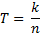Where:

T = number of hours ,   k = constant of proportionality , n = number of people

We know that t = 3 when n = 4

We know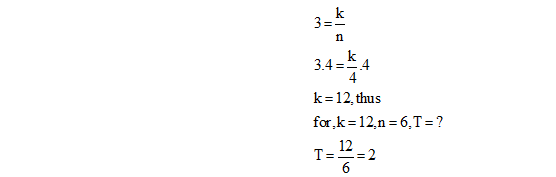By the knowledge of DIRECT PROPORTION,INVERSE PROPORTION we can solve the questions known as Unitary Method.

### Unitary Method

A method in which the value of a unit quantity is first obtained to find the value of any required quantity, is called unitary method.

Unitary Method comprises of following two steps :-

Step 1 = Find the value of one unit.

Step 2 = Then find the value of required units.

Following are some examples explaining Unitary Method.